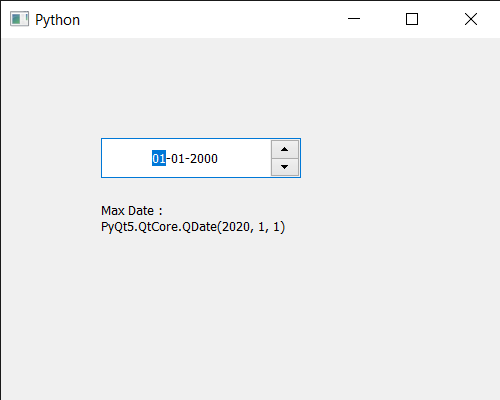# PyQt5 QDateEdit – Getting Maximum Date which user can enter

In this article we will see how we can get the maximum date to the QDateEdit. Sometimes there is a need to set maximum date so that user can’t enter date which is beyond the maximum date for example when user is asked to enter date of birth, user should not be allowed to enter future date then need for setting maximum date arises. Maximum date can be set with the help of `setMaximumDate` method.

In order to do this we use `maximumDate` method with the QDateEdit object

Syntax : date.maximumDate(date)

Argument : It takes no argument

Return : It returns QDate object

Below is the implementation

 `# importing libraries ` `from` `PyQt5.QtWidgets ``import` `*`  `from` `PyQt5 ``import` `QtCore, QtGui ` `from` `PyQt5.QtGui ``import` `*`  `from` `PyQt5.QtCore ``import` `*`  `import` `sys ` ` `  ` `  `class` `Window(QMainWindow): ` ` `  `    ``def` `__init__(``self``): ` `        ``super``().__init__() ` ` `  `        ``# setting title ` `        ``self``.setWindowTitle(``"Python "``) ` ` `  `        ``# setting geometry ` `        ``self``.setGeometry(``100``, ``100``, ``500``, ``400``) ` ` `  `        ``# calling method ` `        ``self``.UiComponents() ` ` `  `        ``# showing all the widgets ` `        ``self``.show() ` ` `  `    ``# method for components ` `    ``def` `UiComponents(``self``): ` ` `  `        ``# creating a QDateEdit widget ` `        ``date ``=` `QDateEdit(``self``) ` ` `  `        ``# setting geometry of the date edit ` `        ``date.setGeometry(``100``, ``100``, ``200``, ``40``) ` ` `  `        ``# alignment ` `        ``a_flag ``=` `Qt.AlignCenter ` ` `  `        ``# setting alignment of date ` `        ``date.setAlignment(a_flag) ` `         `  `        ``# QDate object ` `        ``d ``=` `QDate(``2020``, ``1``, ``1``) ` `         `  `        ``# setting maximum date ` `        ``date.setMaximumDate(d) ` `         `  `         `  `        ``# text ` `        ``text ``=` `"Geek date edit"` `         `  `        ``# setting name ` `        ``date.setAccessibleName(text) ` ` `  `        ``# creating a label ` `        ``label ``=` `QLabel(``"GeeksforGeeks"``, ``self``) ` ` `  `        ``# setting geometry ` `        ``label.setGeometry(``100``, ``150``, ``200``, ``60``) ` ` `  `        ``# making label multiline ` `        ``label.setWordWrap(``True``) ` ` `  `        ``# getting maximum date ` `        ``value ``=` `date.maximumDate() ` ` `  `        ``# setting text to the label ` `        ``label.setText(``"Max Date : "` `+` `str``(value)) ` ` `  ` `  `# create pyqt5 app ` `App ``=` `QApplication(sys.argv) ` ` `  `# create the instance of our Window ` `window ``=` `Window() ` ` `  `# start the app ` `sys.exit(App.``exec``()) `Whether you're preparing for your first job interview or aiming to upskill in this ever-evolving tech landscape, GeeksforGeeks Courses are your key to success. We provide top-quality content at affordable prices, all geared towards accelerating your growth in a time-bound manner. Join the millions we've already empowered, and we're here to do the same for you. Don't miss out - check it out now!

Previous
Next